## 前言

此项目来自于工作需求，起因是我司维护的某款机器一个零件缺货，该零件是用于将摇杆电位器连到机器外操作摇杆的一系列零件中的一个结构件。因该机器是一款儿童玩具，损坏几率不低，没有本零件将无法为其修复。为此计划使用3D打印机制作该零件，且因公司不提供该零件的3D模型文件，为解决此问题，建立本项目。

## 准备

因之前没有接触过3D建模领域，所以该项目查找了大量网络资料。通过查找资料得知，这种工件类设计使用Solidworks（后称SW）比较合适。于是通过查找资料，采用边学习边做的方式尝试对该零件进行建模。

零件形状如图所示;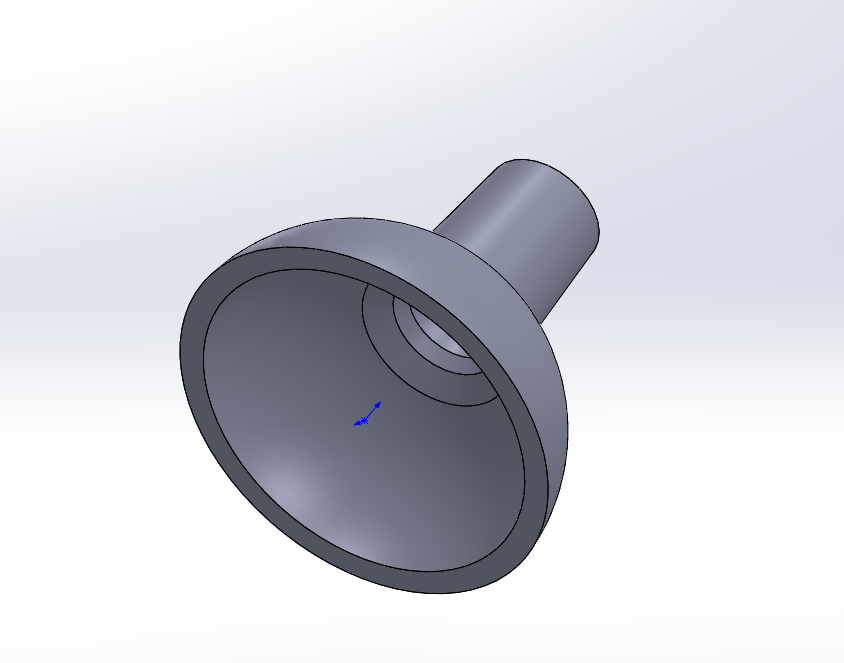考虑零件形状，组成结构类似于一个半球上加一个圆柱，内部是空的，该零件是左右对称的解构。通过搜索引擎得知，SW可以通过草图旋转产生3D模型，于是我们就需要设计一个可以用于旋转产生的草图。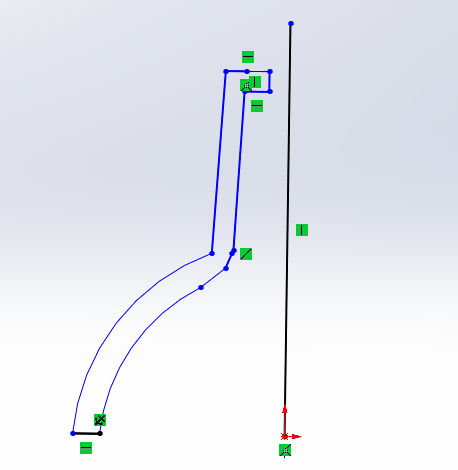接下来事情是要确定下面那个类似半球的结构，其底面直径可以测出，但是观察发现，这个并非一个半球，这个球缺比它所属的球的半球小，这样我们就要先算一下这个球缺所属球体的数据。

## 求解过程

我先画了一个截面图，能够测量出的数据有a = 14.5mm，b = 15.75mm；设r为原始球体的半径，则a+y = r。根据三角行外接圆半径公式
$R = \frac{abc}{4s}$

这个公式中的字母与我图中画的无关，其中R是圆的半径，abc分别为三角形三边的长，S为三角形的面积。如图可见，三角形BCC'是这个圆的内接三角形。

公式套到我的图中就是：$r = \frac{2bcc^′}{4 \frac{2ba}{2}}$ ；下图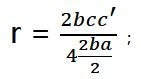代入勾股定理得；
r = (2b(√(a^2+b^2 ))^2)/(4 2ab/2)，下图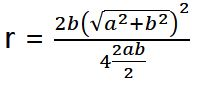化简后得到：
r = (a^2+b^2)/2a，下图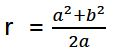将数值代入公式；
r = (14⋅5^2+〖15.75〗^2)/(2×14.5)= 15.8（约值），下图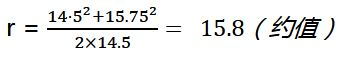至此，我算出了那个球的半径r约为15.8mm
那么，y值约为15.8-14.5 = 1.3mm

# 异或的性质与应用

&#8195今天有朋友问我异或最重要的性质是什么，一时只记得以前有老师讲过，于是上网查了一下，并整理如下。

## 定义

&#8195&#8195异或是一种基于二进制的位运算，用符号XOR或者 ^ 来表示。其运算法则是对运算符两侧的数的每一位二进制位，相同结果为0，不同结果为1。它与或运算的区别在于，当运算符两侧均为1时，或运算结果为1，而异或运算结果为0。
异或运算又称之为 半加 ，半加的概念就是不进位的加法；数学上称异或为 按位模2加 ，按位模2加就是按位进行两数相加后除以2取余的运算。

半加运算是不考虑进位的，也就是说它的进位会被舍弃。很显然它的运算法则如下：
0+1=0 0+1=1 1+0=1 1+1=0
模2运算是一种二进制算法，与四则运算相同。模2运算包含模2加，模2减，模2乘，模2除四种运算。比如模2加，按其定义，1与0模2加，其结果就是1+0 = 1，然后1/2为0余1， 所以1与0模2运算结果为1；再如，1与1模2加，其结果1+1 = 10=2（D），2/2结果为1余0，所以1与1模2加运算结果为0。以此类推，按位模2加的运算结果与半加运算是相同的。

## 性质

1、 交换律
2、 结合律（即(A^B)^C=A^(B^C)）
3、 对于任何数X，都有X^X = 0，X^0 = X
4、 自反性 A^B^B = A^0 = A

异或运算常见于多项式除法，但它最重要的性质是自反性，即A^B^B = A。即对于既定的数A，用同样的运算因子（B）与其做两次异或运算后得到的结果仍是A本身。这是一个神奇的性质，利用这个性质可以解决一些实际问题。例如：几乎所有的C语言教科书都会向初学者提出，要交换两个变量的值，必须引入一个中间变量。但我们知道异或后，我们有了一种新的办法，这种办法可以不使用中间变量。设有A,B两个变量，存储的值分别为a,b，则以下三行表达式可互换他们的值：
A = A^B // (A = a^b)
B = B^A // ( B = b^ (a^b) == a^b^b == a)
A = A^B // ( A = (a^b)^ a == b^a^a == b)

## 应用举例

### 解法一：

显然已经有人提出了一个比较精彩的解法，将所有数加起来，减去1+2+...+1000的和。这个算法已经足够完美了，相信出题者的标准答案也就是这个算法，唯一的问题是，如果数列过大，则可能会导致溢出。

### 解法二：

异或就没有这个问题，并且性能更好。
将所有的数全部异或，得到的结果与1^2^3^...^1000的结果进行异或，最终得到的结果就是重复的数。

这个算法虽然看起来简单，但证明起来并不是一件容易的事情。这与异或运算的几个特性有关：
首先是异或运算满足交换律、结合律。所以，1^2^...^n^...^n^...^1000，无论这两个n出现在什么位置，都可以转换成为1^2^...^1000^(n^n)的形式。
其次，对于任何数x，都有x^x=0，x^0=x。

1^2^...^n^...^n^...^1000 = 1^2^...^1000^(n^n)= 1^2^...^1000^0 = 1^2^...^1000（即序列中除了n的所有数的异或）。

设，1^2^...^1000（该序列中不包含n）的结果为T
则，1^2^...^1000（该序列中包含n）的结果就是T^n
T^(T^n)=n

# DOS指令Attrib的使用方法

attrib指令用于修改文件的属性。文件的常见属性有：只读、存档、隐藏和系统。

attrib指令的格式和常用参数为：

ATTRIB [+R | -R] [+A | -A ] [+S | -S] [+H | -H] [[drive:] [path] filename] [/S [/D]]

+ 设置属性。

– 清除属性。

R 只读文件属性。

A 存档文件属性。

S 系统文件属性。

H 隐藏文件属性。

I 无内容索引文件属性。

[drive:][path][filename]

/S 处理当前文件夹及其子文件夹中的匹配文件。

/D 处理文件夹。

/L 处理符号链接和符号链接目标的属性。

## 举两个例子:

### 1：我要将U盘（盘符G:）根目录一个名为IMG.zip的文件添加只读、系统、隐藏属性。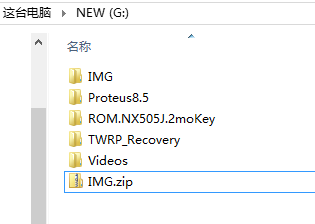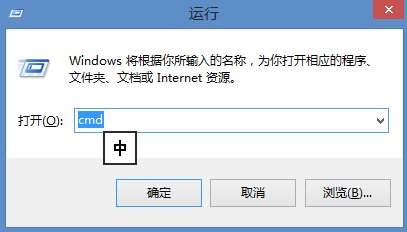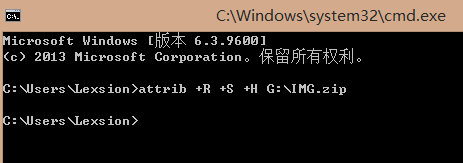U盘中的IMG.zip已经不见了。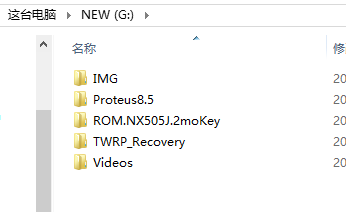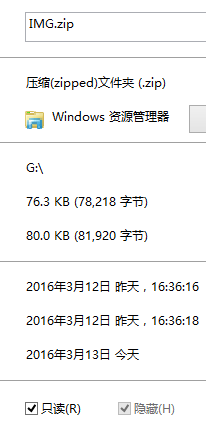### 2：下面的例子，将U盘（盘符G:）根目录的IMG文件夹设置系统 隐藏属性。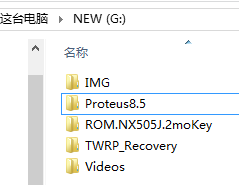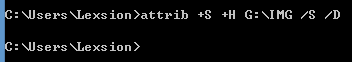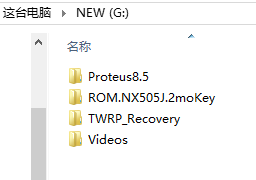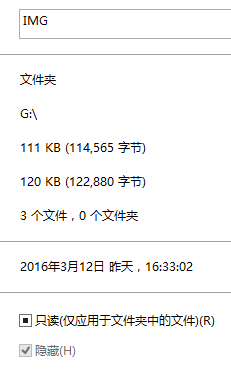## 在VMware Workstation虚拟机中安装Windows7

(相关镜像可以在MSDN I tell You找到)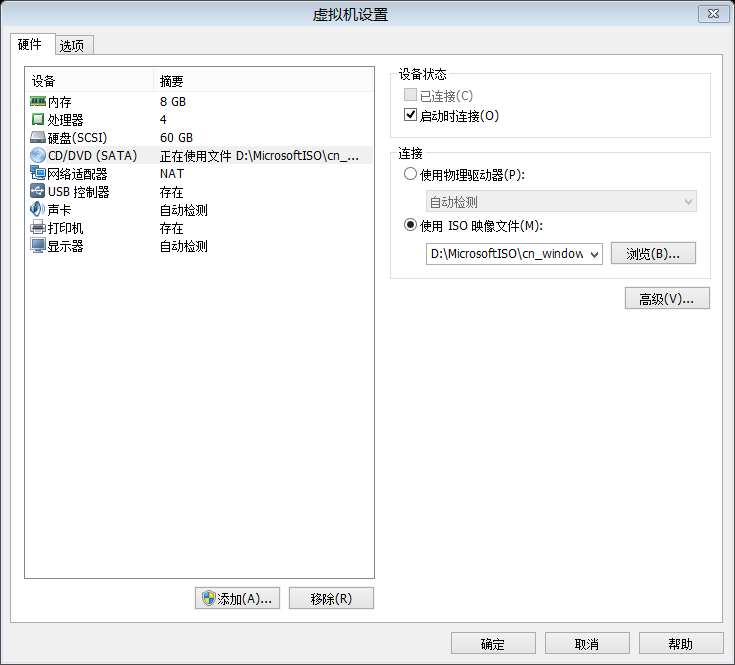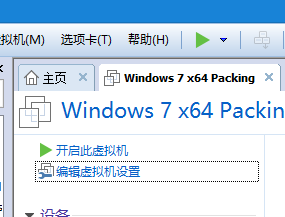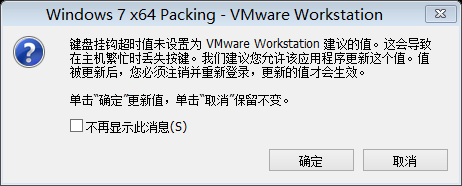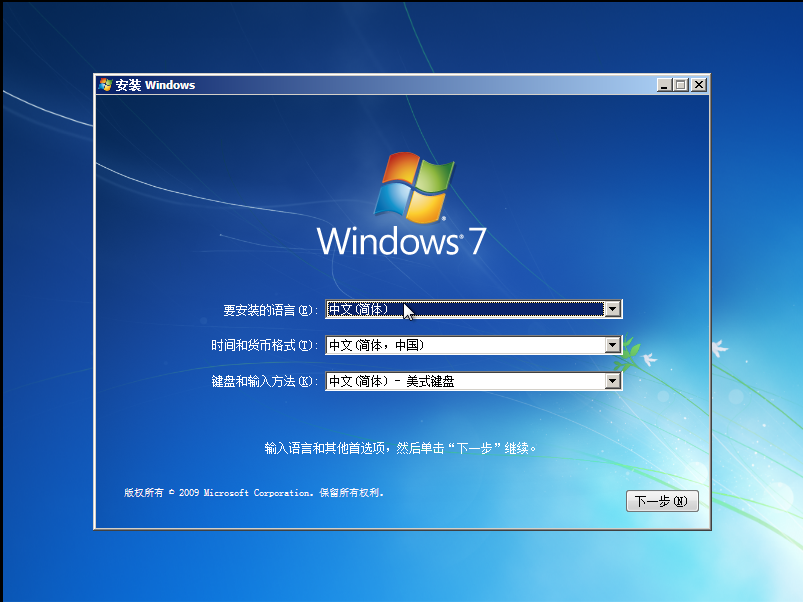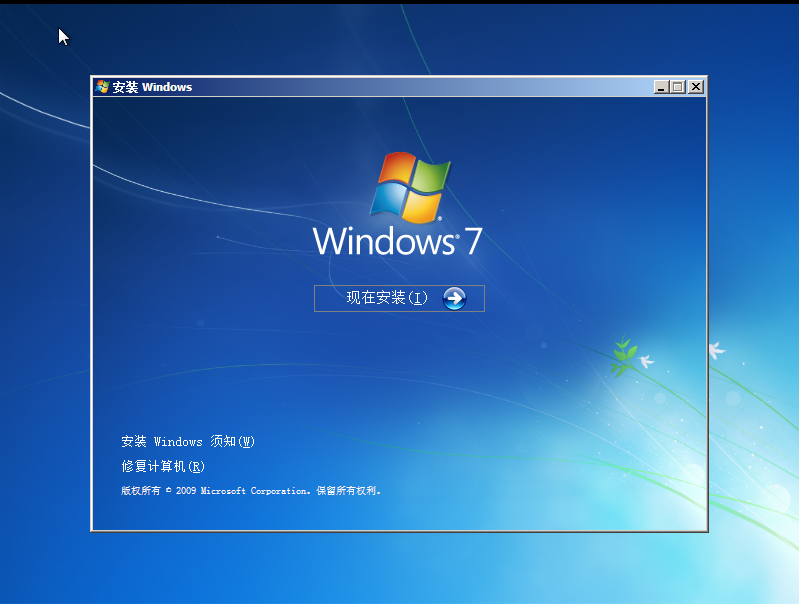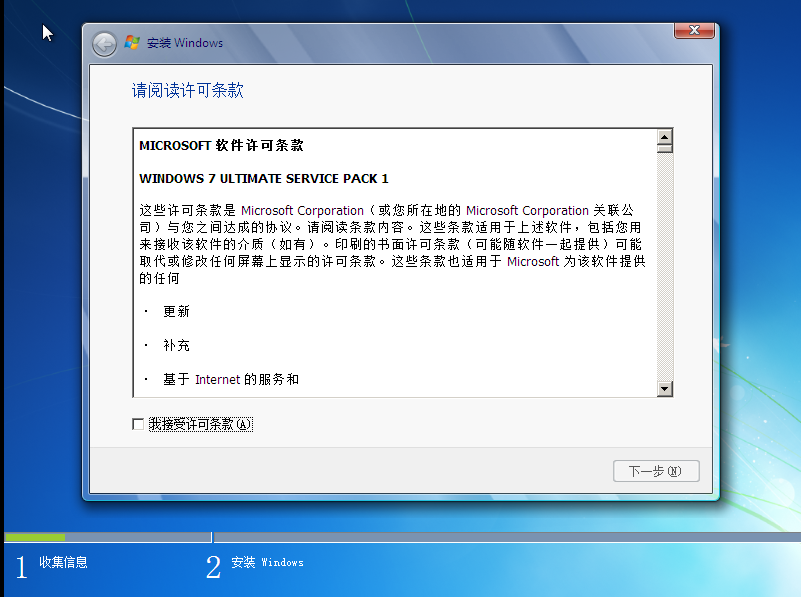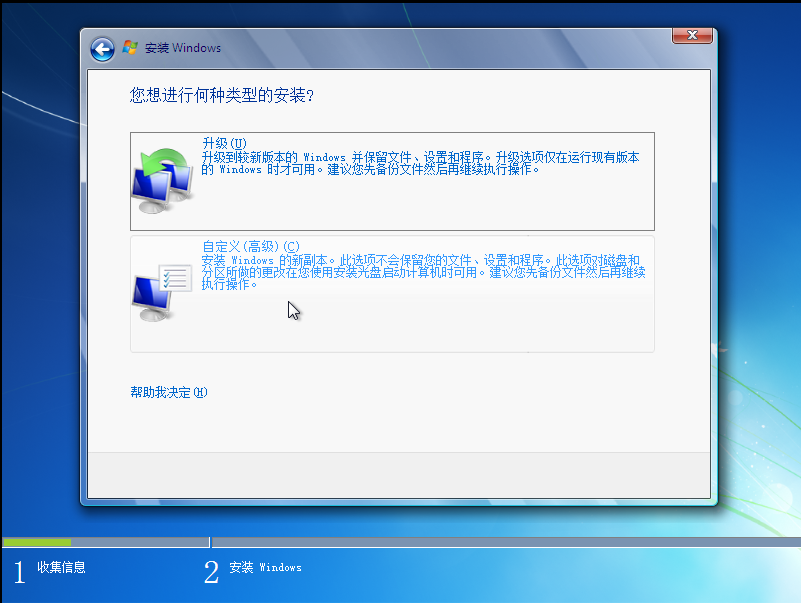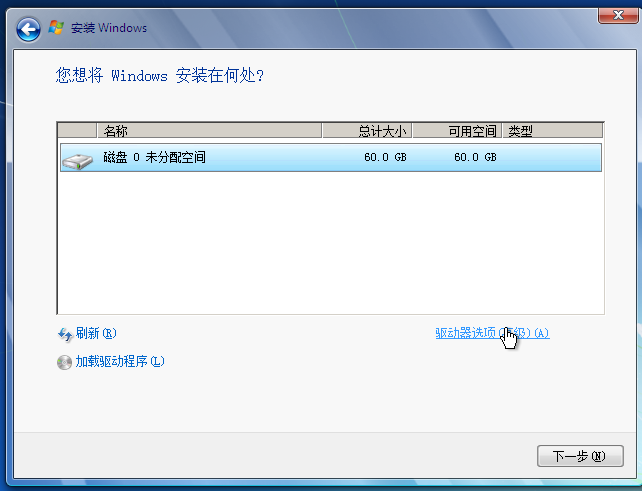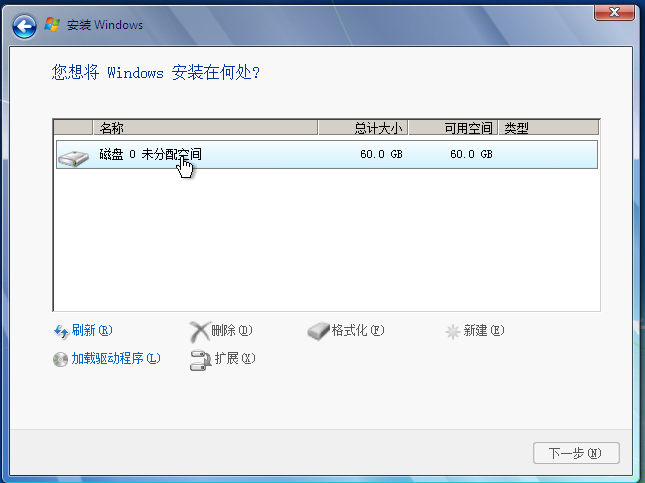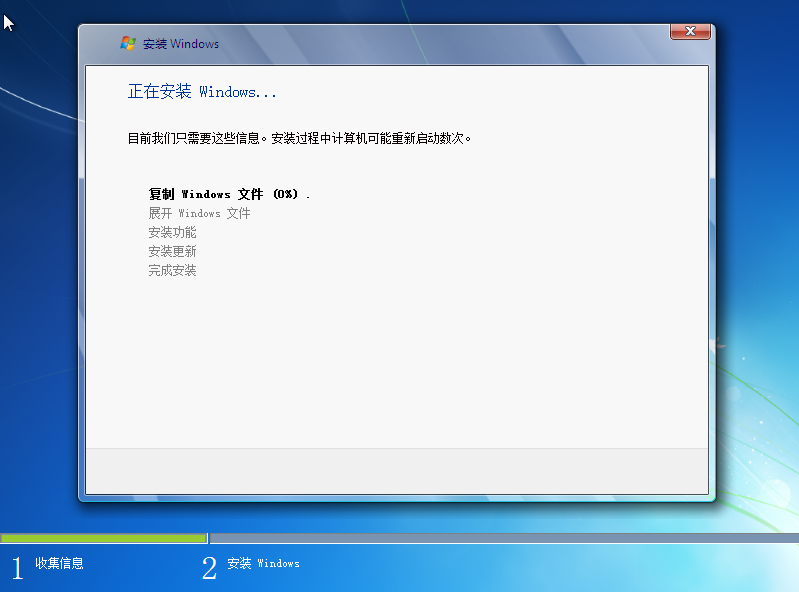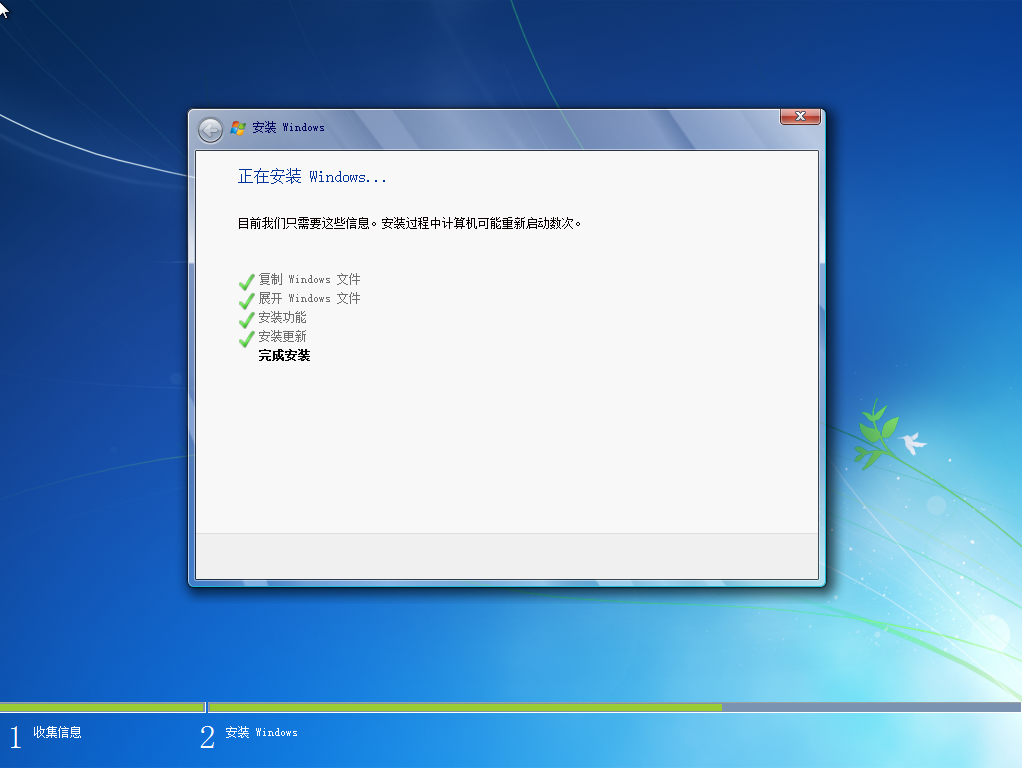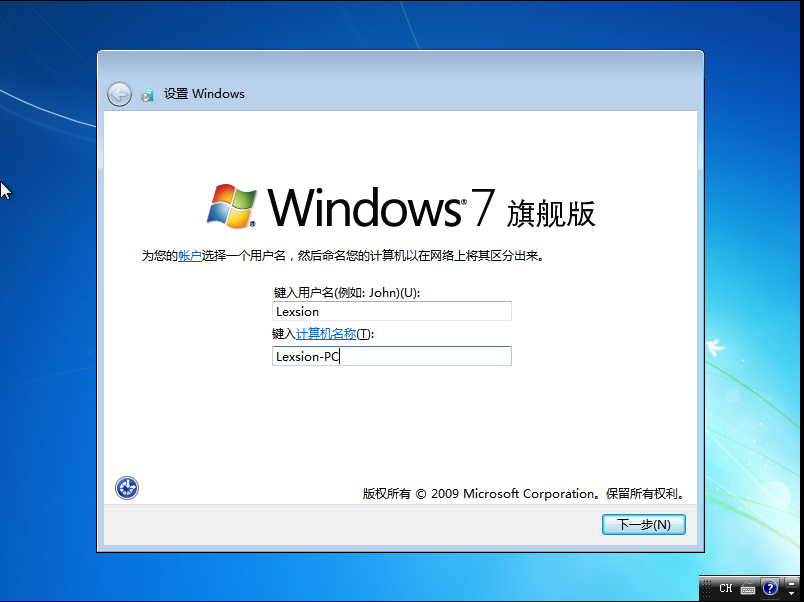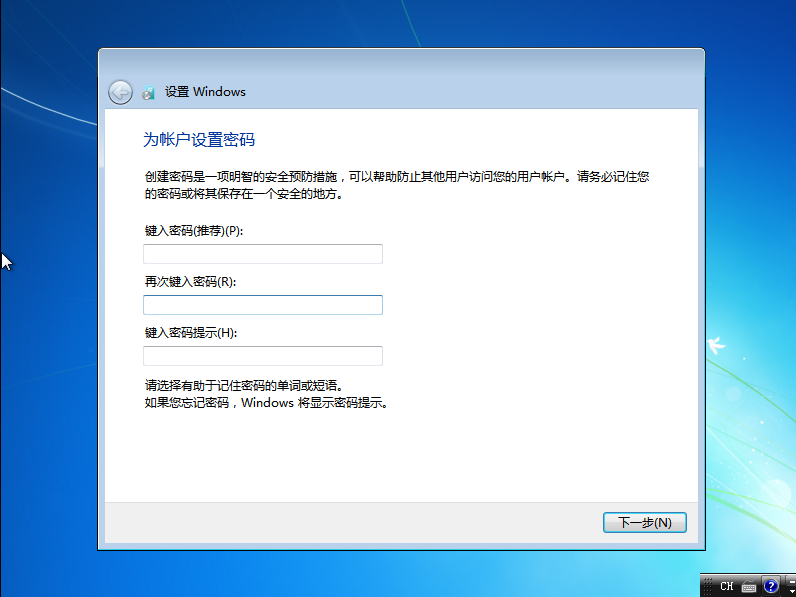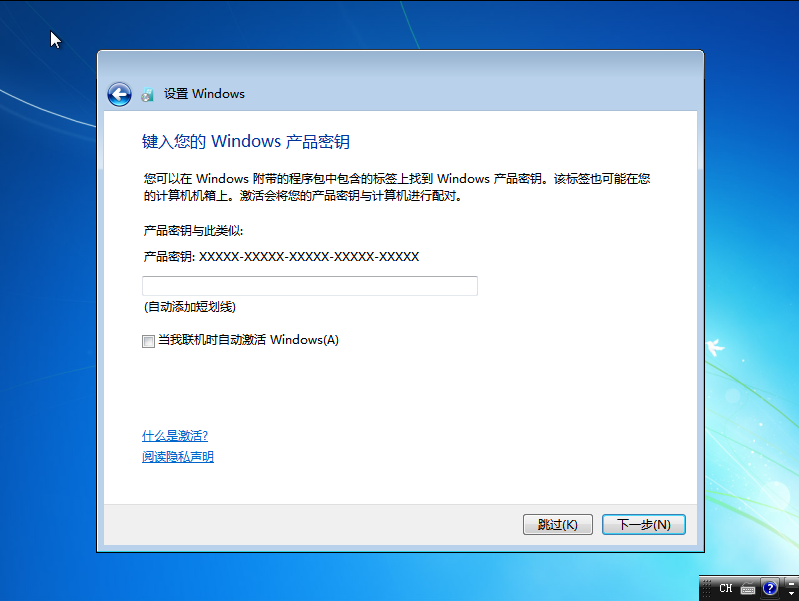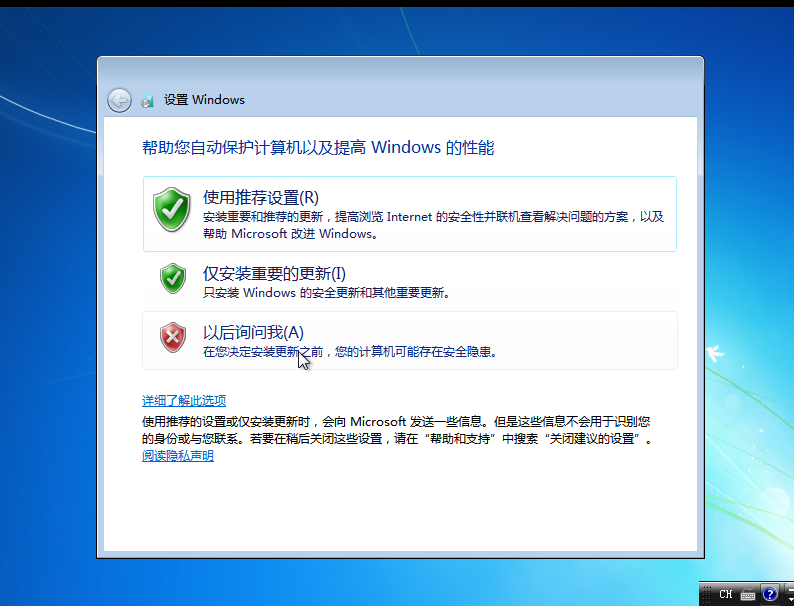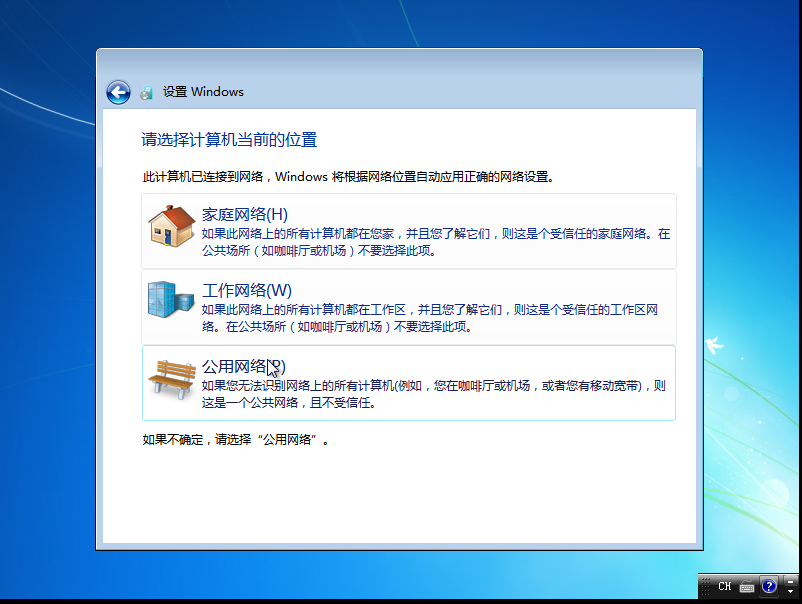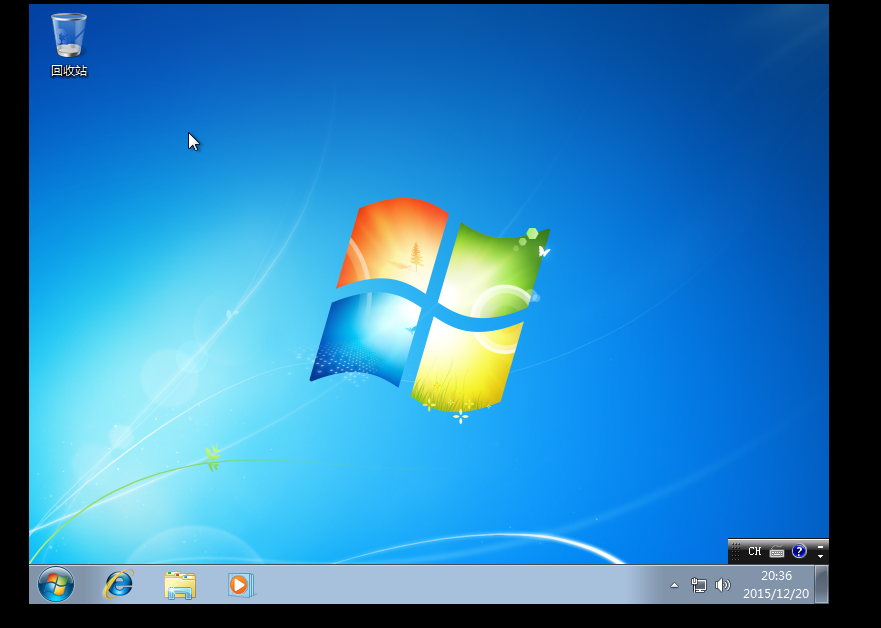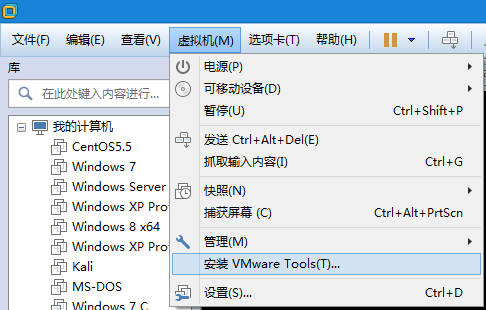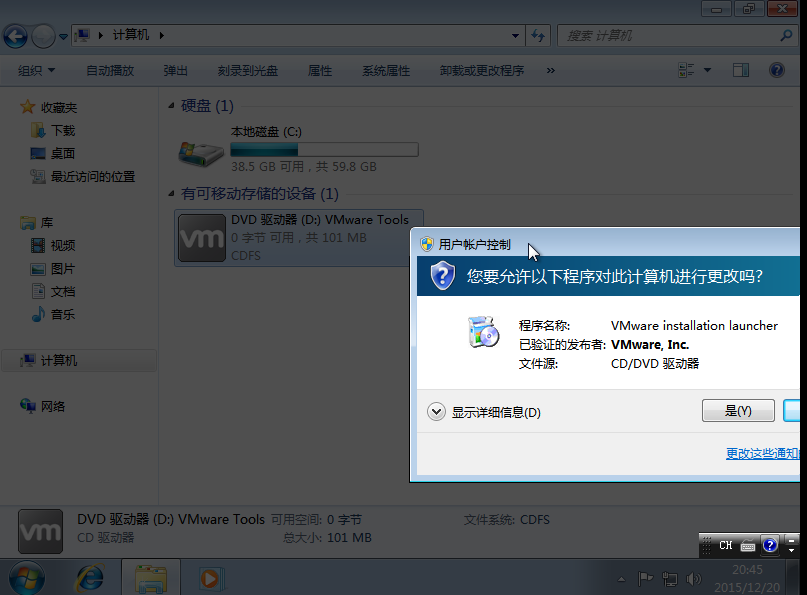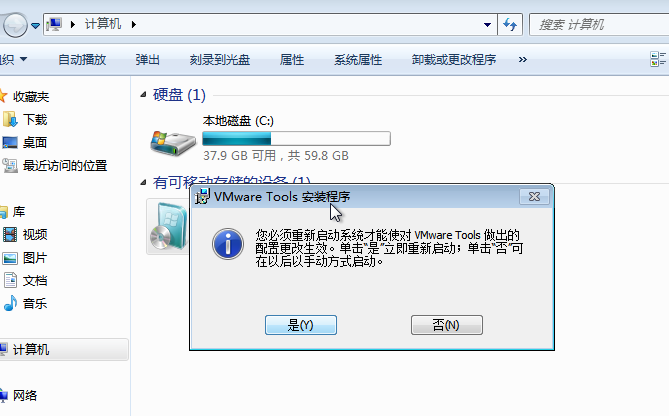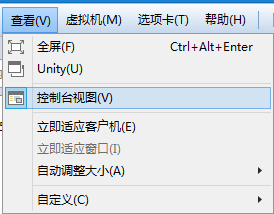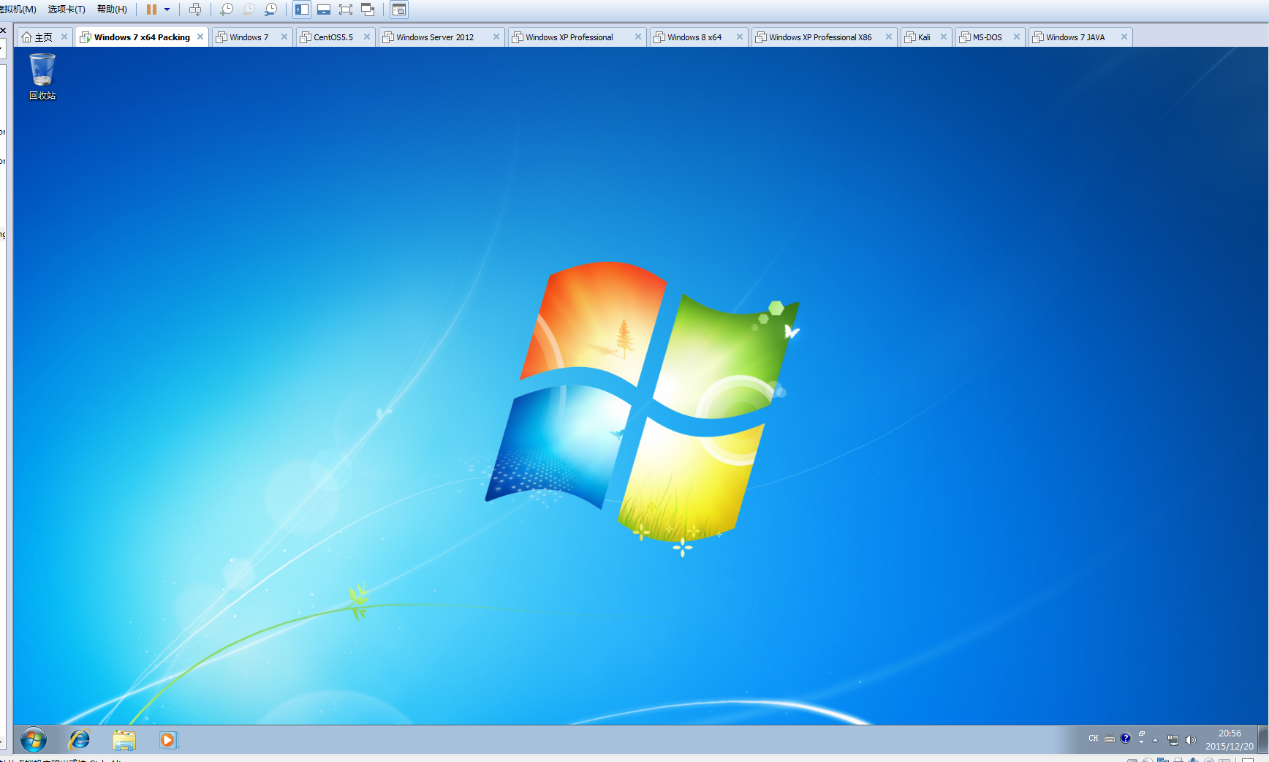## VMware Workstation虚拟机文件的创建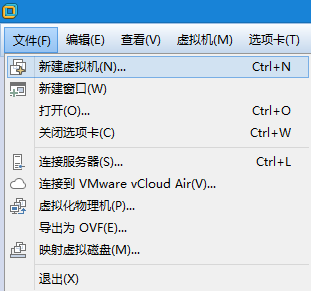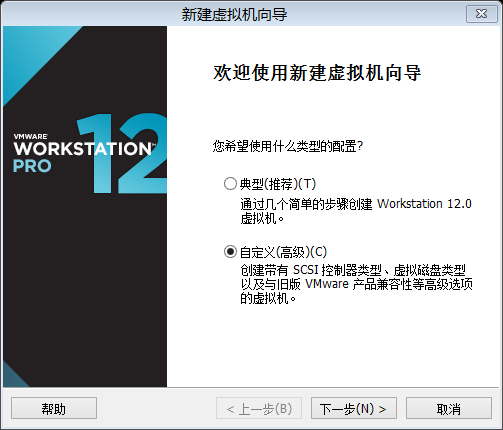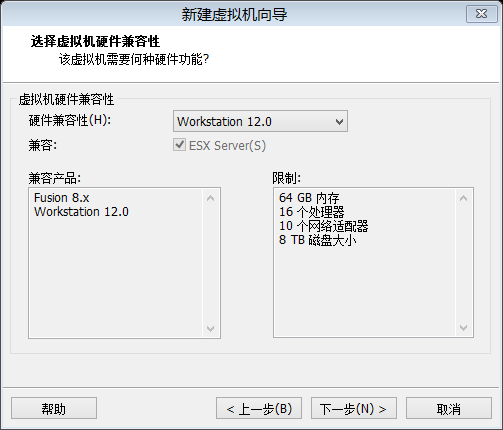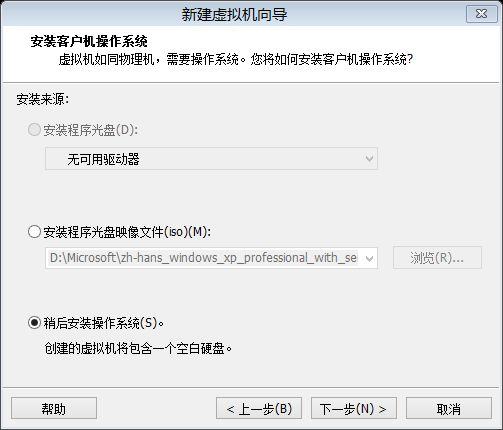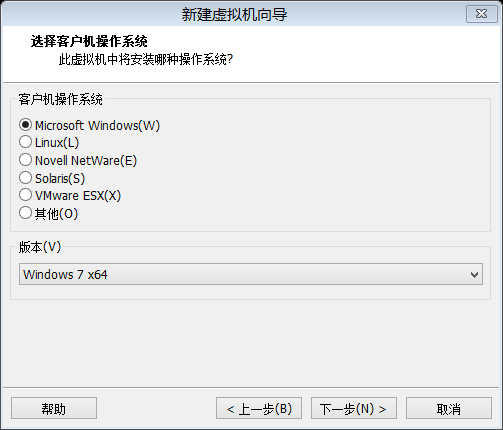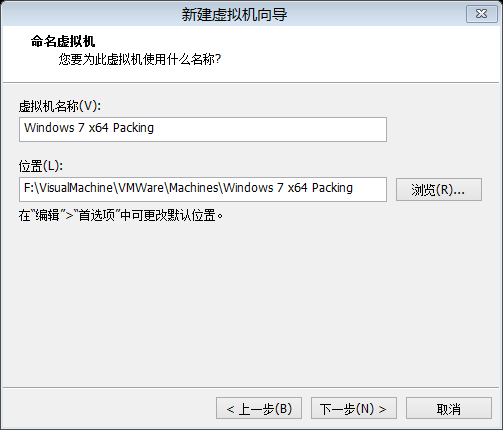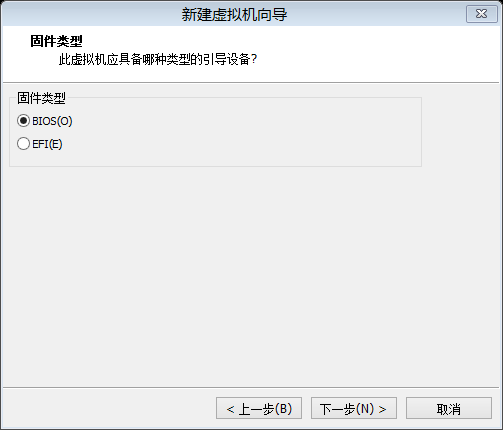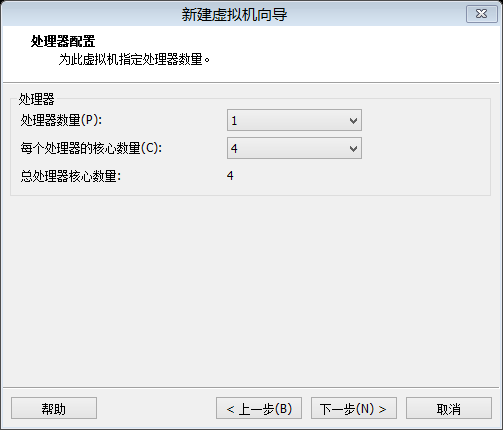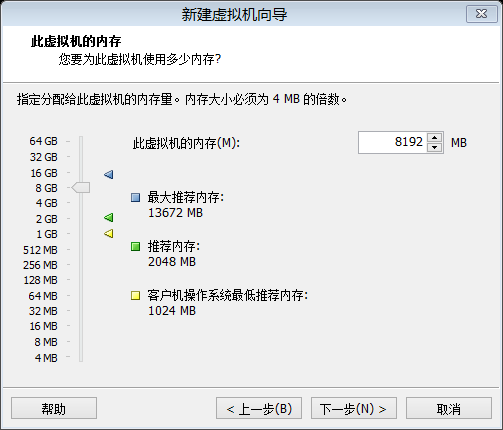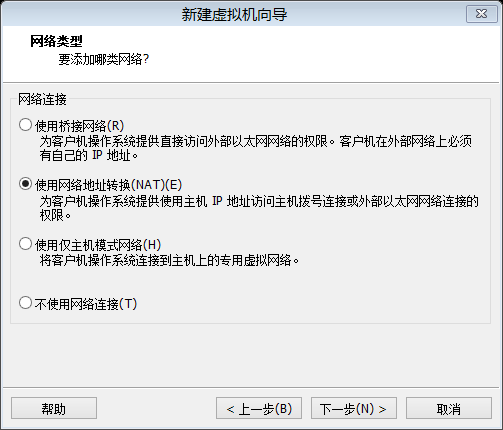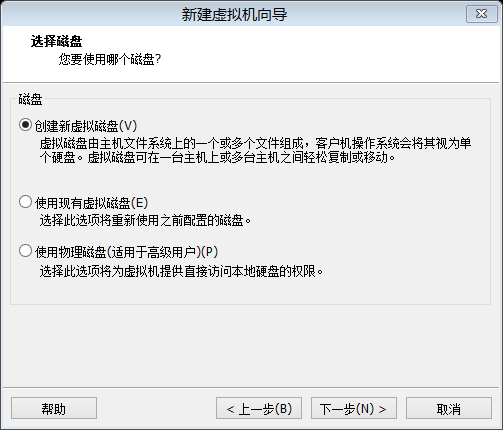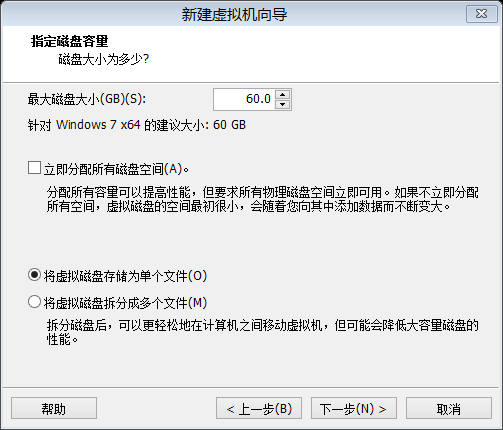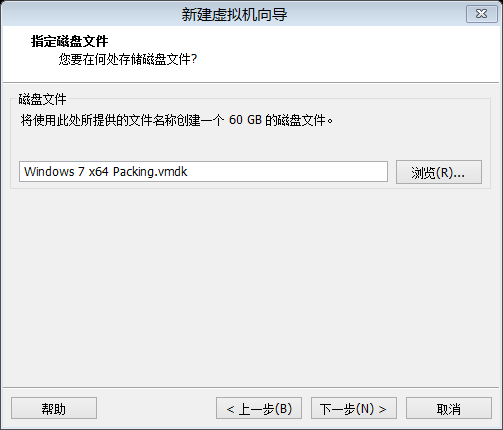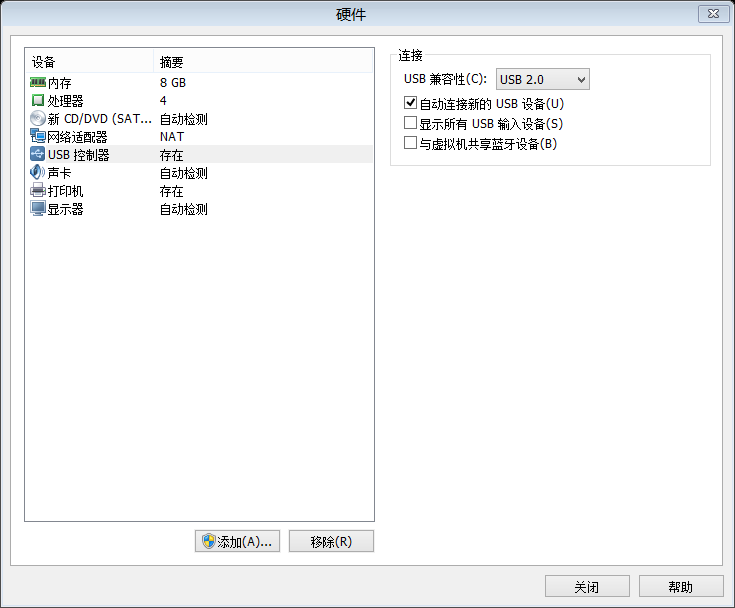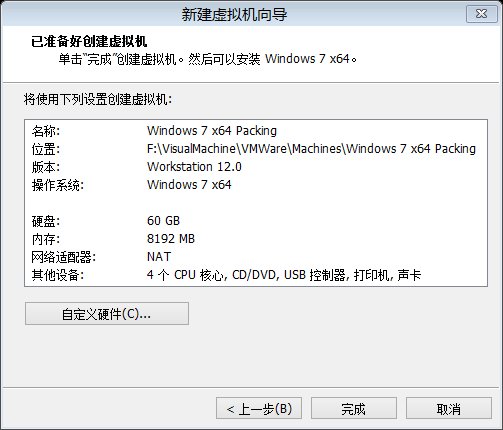## 处理过程：

### 1. 确认中毒情况：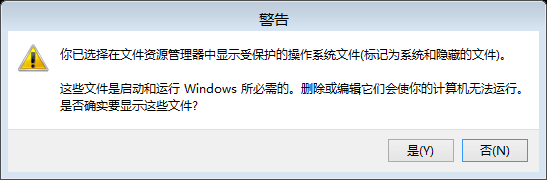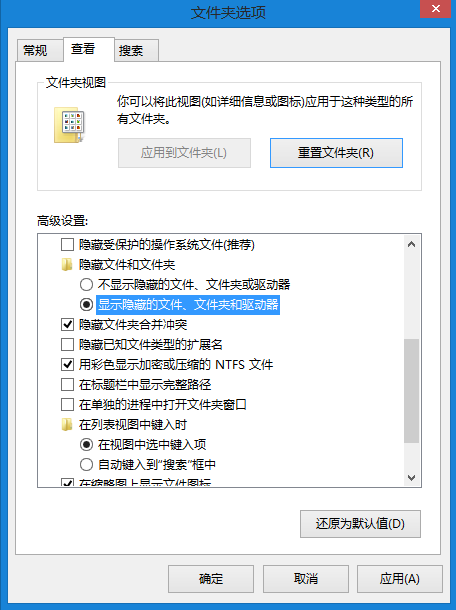### 处理办法：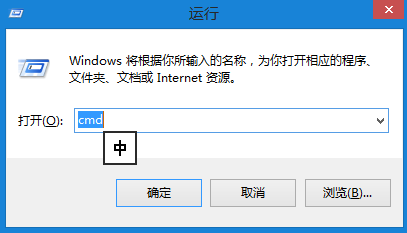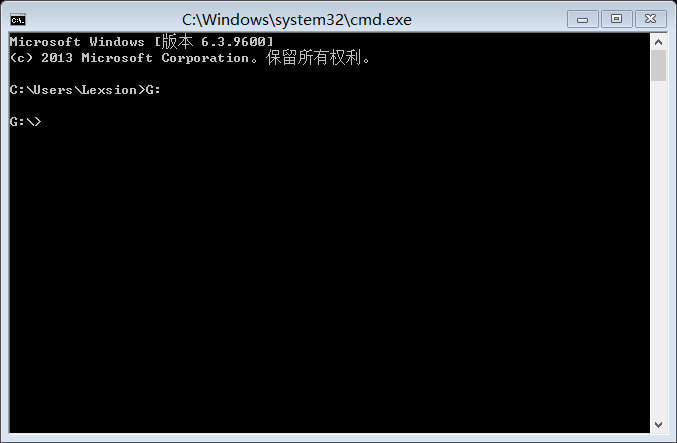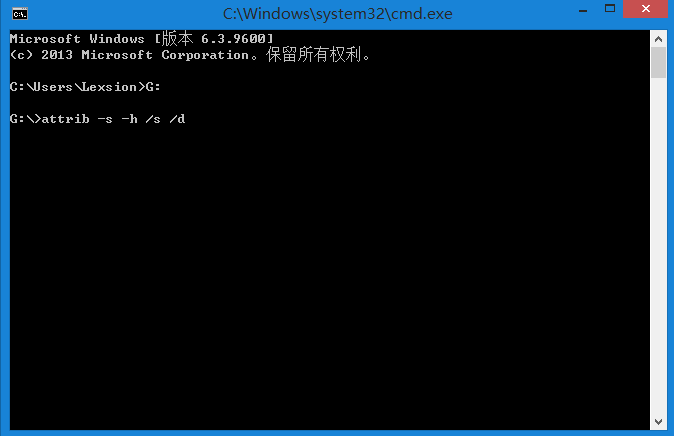Lexsion原创文章，转载请注明出处：
Qzone：https://user.qzone.qq.com/168243371/